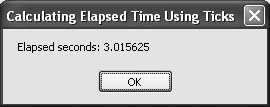# Recipe 7.5. Calculating Elapsed Time Using Ticks

#### Problem

You want a simple way to determine elapsed time when millisecond accuracy is not required.

#### Solution

Sample code folder: Chapter 07\ ElapsedTicks

Use the difference between system ticks returned by Now.Ticks and divide by 10 million to get elapsed decimal seconds.

#### Discussion

As shown in Recipe 7.1, Ticks returns the number of 100-nanosecond time intervals elapsed since midnight of January 1, 1 AD. Dividing Ticks by 10,000,000 converts the time units to seconds. The following code demonstrates this technique by timing how long the user takes to click an OK button and then displaying the number of decimal seconds elapsed:

` Dim ticksBefore As Long Dim ticksAfter As Long Dim tickSeconds As Double ' ----- Time the user! ticksBefore = Now.Ticks MsgBox("Press OK to see elapsed seconds") ticksAfter = Now.Ticks tickSeconds = (ticksAfter - ticksBefore) / 10000000.0 MsgBox("Elapsed seconds: " & tickSeconds.ToString()) `

Figure 7-5 shows the result.

##### Figure 7-5. Using Ticks to measure elapsed decimal secondsThis is a simple technique for getting decimal seconds for each moment in time, but the real workhorse for determining spans of time is the TimeSpan object, which is demonstrated in Recipe 7.6.Visual Basic 2005 Cookbook: Solutions for VB 2005 Programmers (Cookbooks (OReilly))
ISBN: 0596101775
EAN: 2147483647
Year: 2006
Pages: 400

Similar book on Amazon